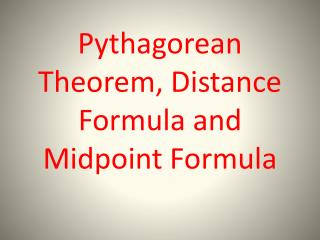# Pythagorean Theorem, Distance Formula and Midpoint Formula - PowerPoint PPT PresentationDownload PresentationPythagorean Theorem, Distance Formula and Midpoint Formula

Download Presentation## Pythagorean Theorem, Distance Formula and Midpoint Formula

- - - - - - - - - - - - - - - - - - - - - - - - - - - E N D - - - - - - - - - - - - - - - - - - - - - - - - - - -
##### Presentation Transcript

1. Pythagorean Theorem, Distance Formula and Midpoint Formula

2. Pythagorean Theorem

3. Pythagorean Theorem Word Problems • A square has a diagonal with length of 20 cm. What is the measure of each side? Round to the nearest tenths.

4. Pythagorean Theorem Word Problems • A 25 foot ladder is leaning against a building. The foot of the ladder is 15 feet from the base of the building. How high is the top of the ladder along the building? Round to the nearest tenths.

5. Pythagorean Theorem Word Problems • Ashley travels 42 miles east, then 19 miles south. How far is Ashley from the starting point? Round to the nearest tenths.

6. Pythagorean Theorem Word Problems • What is the length of the altitude of an equilateral triangle if a side is 12 cm? Round to the nearest tenths.

7. The Distance Formula Given two points:

8. Example Find the distance between (1, 4) and (-2, 3). Round to the nearest hundredths. D 3.16

9. Example Find the distance between the points, (10, 5) and (40, 45). Round to the nearest hundredths. D = 50

10. 3. Find the distance between the points. Round to the nearest tenths. 3.6

11. 4. Find the distance between the points. Round to the nearest tenths. 3.2

12. Midpoint Given 2 ordered pairs, it’s the AVG of the x’s and AVG of the y’s.

13. Midpoint Formula

14. Find the midpoint. 1. (3, 7) and (-2, 4) 2. (5, -2) and (6, 14) (.5, 5.5) (5.5, 6)

15. Find the midpoint. 3. (3, -9) and (14, 16) 4. (12, 17) and (-7, 9) (8.5, 3.5) (2.5, 13)

16. Find the midpoint. 5. (2, 3)

17. Find the midpoint. 6. (1.5, 4)

18. Given the midpt and one endpt, find the other endpt. 7. Midpt(3, -6) Endpt(7, -3) (-1, -9)

19. Given the midpt and one endpt, find the other endpt. 8. Midpt(-1, 2) Endpt(3, 0) (-5, 4)

20. Given the midpt and one endpt, find the other endpt. 9. Midpt(-4, 6) Endpt(2, 1) (-10, 11)